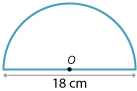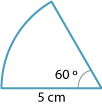#### Question 1

 The area of a circle with radius 6 cm is: 12π cm² 24π cm² 36π cm²

#### Question 2

 The area of a circle with diameter 8 cm is: 16π cm² 36π cm² 64π cm²

#### Question 3The area of the shape pictured is: 36π cm² $$\dfrac{81\pi}{2}\ \text{cm}^2$$ 162π cm²

#### Question 4

 If the diameter of a circle is doubled, then its area is: divided by two multiplied by two multiplied by four

#### Question 5The area of the sector shown is: $$\dfrac{5π}{3}\ \text{cm}^2$$ $$\dfrac{25π}{6}\ \text{cm}^2$$ $$\dfrac{25π}{4}\ \text{cm}^2$$